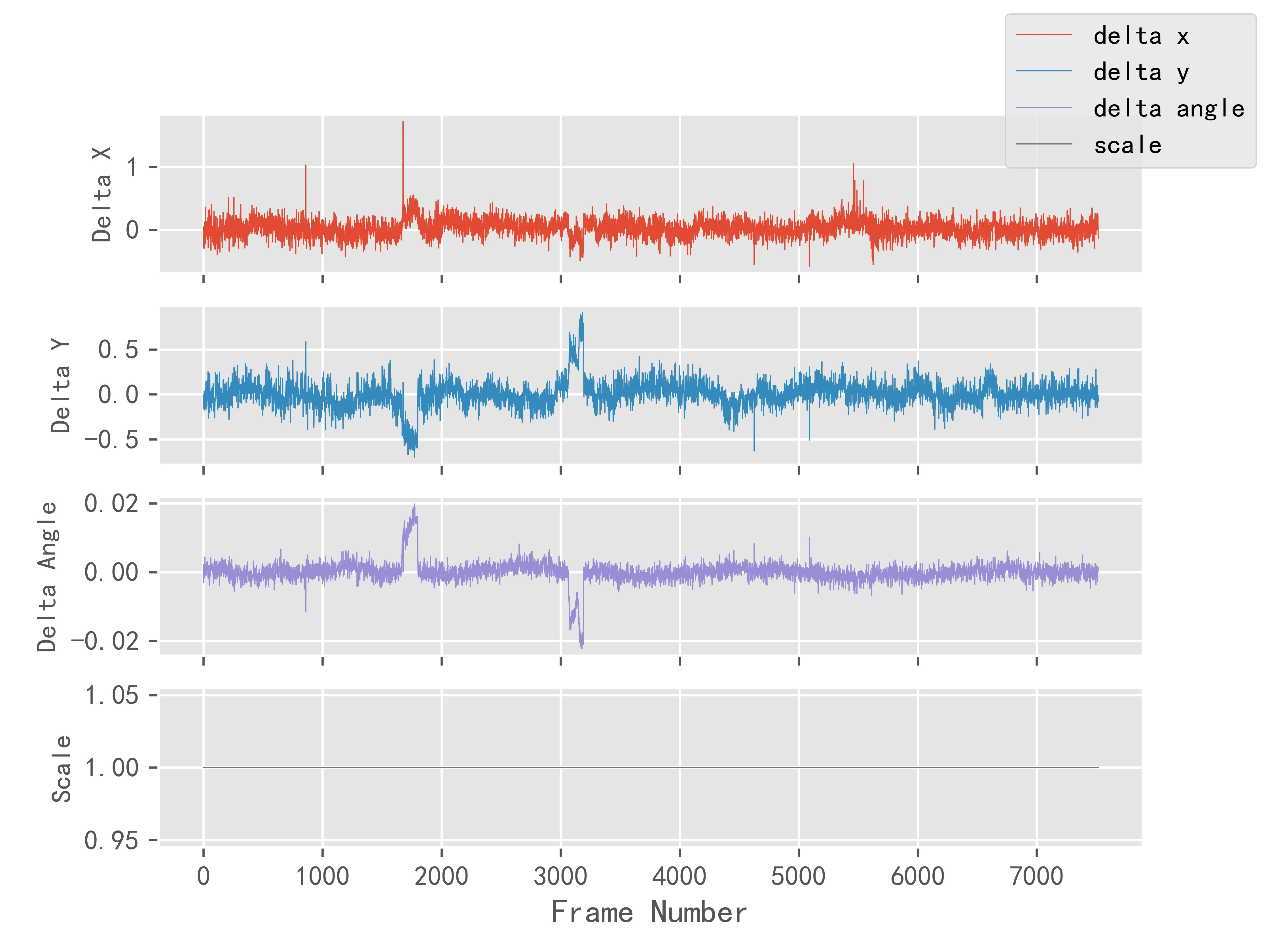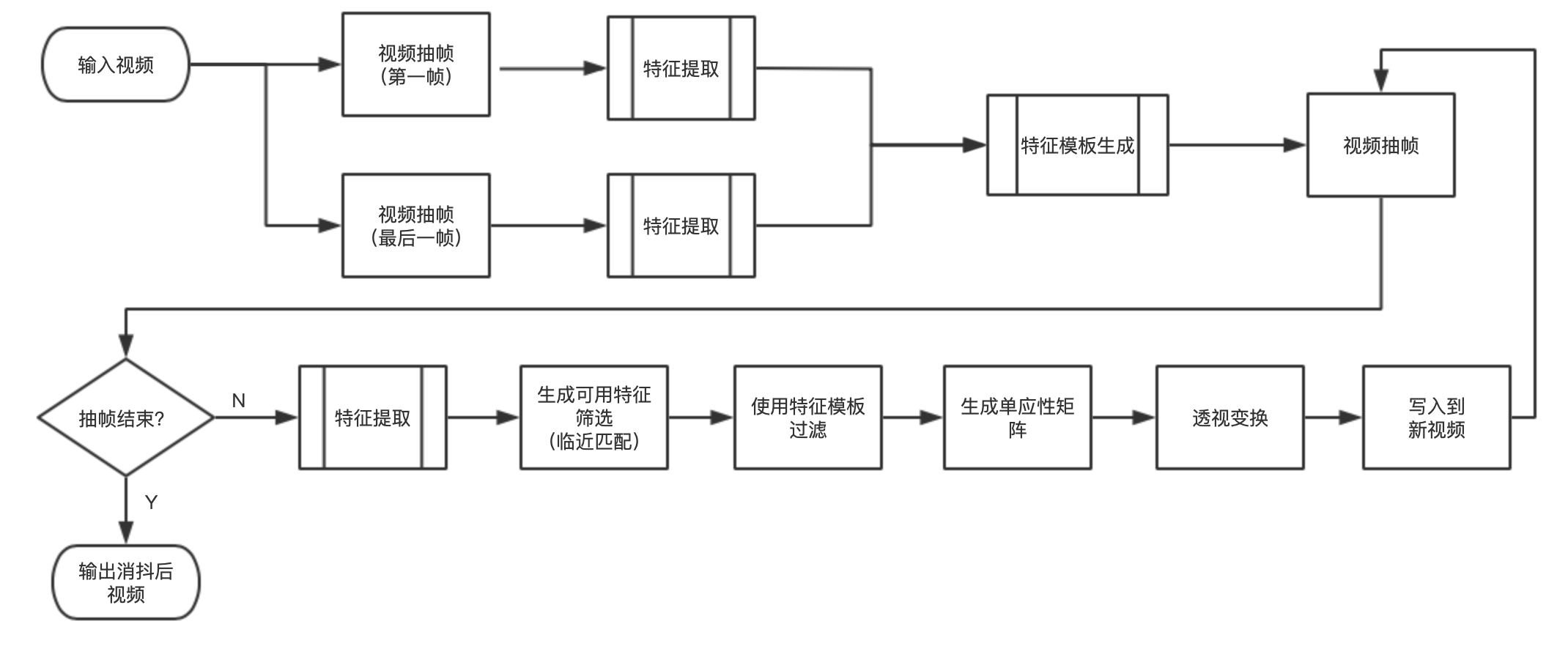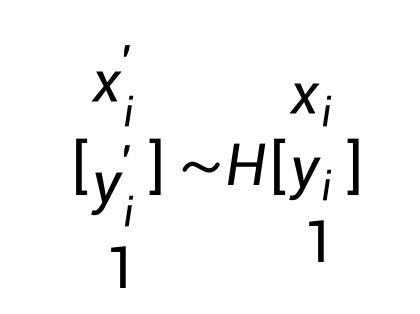## 基于opencv对高空拍摄视频消抖处理_opencv 视频防抖_js君的博客-程序员秘密

### 二、量化指标### 三、技术思路（1）首先对视频进行抽第一帧与最后一帧，为什么抽取两帧？这样做的主要目的是，我们在做帧对齐时，使用帧中静态物的关键点做对齐，如果特征点来源于动态物上，那么对齐后就会产生形变，我们选取第一帧与最后一帧，提取特征点，留下交集部分，则可以得到静态特征点我们这里称为特征模板，然后将特征模板应用到每一帧上，这样可以做有效对齐。

（2）常用特征点检测器：

SIFT: 04 年提出，广泛应用于各种跟踪和识别算法，表现能力强，但计算复杂度高。

SURF: 06 年提出，是 SIFT 的演进版本，保持强表现能力的同时大大减少了计算量。

BRISK: BRIEF 的演进版本，压缩了特征的表示，提高了匹配速度。 ORB: 以速度著称，是 SURF 的演进版本，多用于实时应用。

GFTT: 最早提出的 Harris 角点的改进版本，经常合称为 Harris-Shi-Tomasi 角点。

SimpleBlob: 使用 blob 的概念来抽取图像中的特征点，相对于角点的一种创新。 FAST: 相比其他方法特征点数量最多，但也容易得到距离过近的点，需要经过 NMS。

Star: 最初用于视觉测距，后来也成为一种通用的特征点检测方法。

（3）在上图中，我们发现所提取的特征点中部分来自于车身，由于车是运动的，所以我们不能使用，我们用第一帧与最后一帧做静态特帧点匹配，生成静态特征模板，在下图中，我们发现只有所有的特征点只选取在静态物上

（4）静态特征模板匹配 ，我们这里使用Flann算法，匹配结果如下

（5）使用匹配成功的两组特征点，估计两帧之间的透视变换 (Perspective Transformation)。估计矩阵 H，其中 (x_i, y_i) 和 (x_i^′, y_i^′) 分别是两帧的特征点。### 四、实现代码

python版本：3.X
opencv-python：3.4.2.16
opencv-contrib-python：3.4.2.16

``````需要卸载之前的opencv-python版本
pip uninstall opencv-python
pip uninstall opencv-contrib-python

pip install opencv_python==3.4.2.16
pip install opencv-contrib-python==3.4.2.16``````

``````import cv2
import numpy as np
from tqdm import tqdm
import argparse
import os

# get param
parser = argparse.ArgumentParser(description='')
parser.add_argument('-n', type=int, default=-1)  # 指定处理的帧数（参数可选）, 不设置使用视频实际帧

# eg: python3 stable.py -v=video/01.mp4 -o=video/01_stable.mp4 -n=100 -p=6

args = parser.parse_args()

input_path = args.v
output_path = args.o
number = args.n

class Stable:
# 处理视频文件路径
__input_path = None

__output_path = None

__number = number

# surf 特征提取
__surf = {
# surf算法
'surf': None,
# 提取的特征点
'kp': None,
# 描述符
'des': None,
# 过滤后的特征模板
'template_kp': None
}

# capture
__capture = {
# 捕捉器
'cap': None,
# 视频大小
'size': None,
# 视频总帧
'frame_count': None,
# 视频帧率
'fps': None,
# 视频
'video': None,
}

# 配置
__config = {
# 要保留的最佳特征的数量
'key_point_count': 5000,
# Flann特征匹配
'index_params': dict(algorithm=0, trees=5),
'search_params': dict(checks=50),
'ratio': 0.5,
}

# 特征提取列表
__surf_list = []

def __init__(self):
pass

# 初始化capture
def __init_capture(self):
self.__capture['cap'] = cv2.VideoCapture(self.__video_path)
self.__capture['size'] = (int(self.__capture['cap'].get(cv2.CAP_PROP_FRAME_WIDTH)),
int(self.__capture['cap'].get(cv2.CAP_PROP_FRAME_HEIGHT)))

self.__capture['fps'] = self.__capture['cap'].get(cv2.CAP_PROP_FPS)

self.__capture['video'] = cv2.VideoWriter(self.__output_path, cv2.VideoWriter_fourcc(*"mp4v"),
self.__capture['fps'], self.__capture['size'])

self.__capture['frame_count'] = int(self.__capture['cap'].get(cv2.CAP_PROP_FRAME_COUNT))

if number == -1:
self.__number = self.__capture['frame_count']
else:
self.__number = min(self.__number, self.__capture['frame_count'])

# 初始化surf
def __init_surf(self):

self.__capture['cap'].set(cv2.CAP_PROP_POS_FRAMES, 0)

self.__capture['cap'].set(cv2.CAP_PROP_POS_FRAMES, self.__capture['frame_count'] - 1)

self.__surf['surf'] = cv2.xfeatures2d.SURF_create(self.__config['key_point_count'])

self.__surf['kp'], self.__surf['des'] = self.__surf['surf'].detectAndCompute(first_frame, None)
kp, des = self.__surf['surf'].detectAndCompute(last_frame, None)

# 快速临近匹配
flann = cv2.FlannBasedMatcher(self.__config['index_params'], self.__config['search_params'])
matches = flann.knnMatch(self.__surf['des'], des, k=2)

good_match = []
for m, n in matches:
if m.distance < self.__config['ratio'] * n.distance:
good_match.append(m)

self.__surf['template_kp'] = []
for f in good_match:
self.__surf['template_kp'].append(self.__surf['kp'][f.queryIdx])

# 释放
def __release(self):
self.__capture['video'].release()
self.__capture['cap'].release()

# 处理
def __process(self):

current_frame = 1

self.__capture['cap'].set(cv2.CAP_PROP_POS_FRAMES, 0)

process_bar = tqdm(self.__number, position=current_frame)

while current_frame <= self.__number:
# 抽帧

if not success: return

# 计算
frame = self.detect_compute(frame)

# 写帧
self.__capture['video'].write(frame)

current_frame += 1

process_bar.update(1)

# 视频稳像
def stable(self, input_path, output_path, number):
self.__video_path = input_path
self.__output_path = output_path
self.__number = number

self.__init_capture()
self.__init_surf()
self.__process()
self.__release()

# 特征点提取
def detect_compute(self, frame):

frame_gray = cv2.cvtColor(frame, cv2.COLOR_BGR2GRAY)

# 计算特征点
kp, des = self.__surf['surf'].detectAndCompute(frame_gray, None)

# 快速临近匹配
flann = cv2.FlannBasedMatcher(self.__config['index_params'], self.__config['search_params'])
matches = flann.knnMatch(self.__surf['des'], des, k=2)

# 计算单应性矩阵
good_match = []
for m, n in matches:
if m.distance < self.__config['ratio'] * n.distance:
good_match.append(m)

# 特征模版过滤
p1, p2 = [], []
for f in good_match:
if self.__surf['kp'][f.queryIdx] in self.__surf['template_kp']:
p1.append(self.__surf['kp'][f.queryIdx].pt)
p2.append(kp[f.trainIdx].pt)

# 单应性矩阵
H, _ = cv2.findHomography(np.float32(p2), np.float32(p1), cv2.RHO)

# 透视变换
output_frame = cv2.warpPerspective(frame, H, self.__capture['size'], borderMode=cv2.BORDER_REPLICATE)

return output_frame

if __name__ == '__main__':

if not os.path.exists(input_path):
exit(0)
else:
print(f'[INFO] Video "{input_path}" stable begin')

s = Stable()
s.stable(input_path, output_path, number)

print('[INFO] Done.')
exit(0)
``````

-v    指定输入视频路径位置（参数必选）

-o    指定输出视频路径位置（参数必选）

-n    指定处理的帧数（参数可选）, 不设置使用视频实际帧

python3 stable.py -v=test.mp4 -o=test_stable.mp4

### Python爬虫selenium提取数据之driver对象常用方法_driver.page_source_bfhonor的博客-程序员秘密

selenium提取数据知识点：1. driver对象的常用属性和方法在使用selenium过程中，实例化driver对象后，driver对象有一些常用的属性和方法driver.page_source 当前标签页浏览器渲染之后的网页源代码driver.current_url 当前标签页的urldriver.close() 关闭当前标签页，如果只有一个标签

### name ‘train_test_split‘ is not defined解决方法-程序员秘密

name 'train_test_split' is not defined解决方法

### 【数据聚类】第四章第一节3：DBSCAN性能分析、优缺点和参数选择方法_怎么评价dbscan分类器的性能_快乐江湖的博客-程序员秘密

DBSCAN算法对数据集中的每个点都要检索其邻域内的所有点，时间复杂度为O(n2)O(n^{2})O(n2)。但在低纬空间中，若采用kkk-ddd树、RRR树等结构，可以有效地检索特定点给定距离内的所有点，其时间复杂度可以降低到O(nlogn)O(nlogn)O(nlogn)①：可以对任意形状的稠密数据集进行聚类②：可以在聚类的同时发现异常点，对数据集中的异常点不敏感③：不需要指定簇数，并且多次实验结果往往是相同的①：如果样本集的密度不均匀、聚类间距差相差很大时，聚类质量较差，这时用DBSCAN聚类一般不适

### java.lang.NoClassDefFoundError: org/apache/ibatis/session/SqlSessionFactory_翱翔天地的博客-程序员秘密

1、错误描述org.springframework.beans.factory.BeanCreationException: Error creating bean with name 'processEngineConfiguration' defined in class path resource [activiti.cfg.xml]: Initialization of bean fail